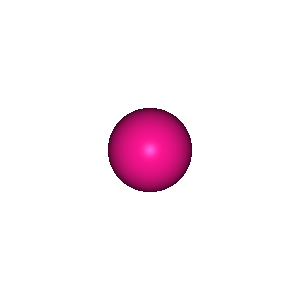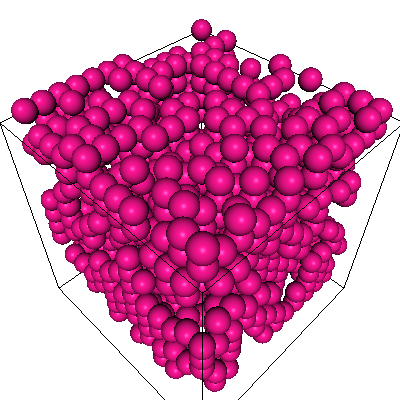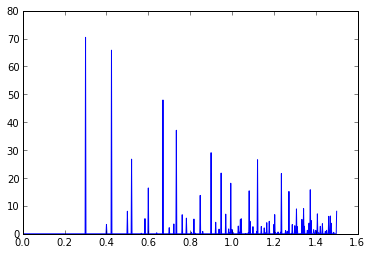# chemlab.ipython demonstration¶

In this notebook we'll see how to use the chemlab.ipython ipython extension to build and calculate the radial distribution function of a random box of Ar.

In :
# Activate the extension

In :
# Imports
from chemlab.db import CirDB
from chemlab.core import random_lattice_box
from chemlab.molsim.analysis import rdf # The path of this function may change in future releases


It is possible to download and display a molecule either by simply evaluate a Molecule instance as the last statement, or by using the function IPython.display.display

In :
ar = CirDB().get("molecule", 'Ar')
ar

Out:The same thing works also for systems.

In :
# Let's make a random box

s = random_lattice_box([ar], , [4.0, 4.0, 4.0])
s

Out:In :
# Let's calculate the radial distribution function
help(rdf)

Help on function rdf in module chemlab.molsim.analysis:

rdf(coords_a, coords_b, binsize=0.002, cutoff=1.5, periodic=None, normalize=True)
Calculate the radial distribution function of *coords_a* against
*coords_b*.

**Parameters**
- coords_a: np.ndarray((3, NA))
first set of coordinates
- coords_b: np.ndarray((3, NB))
coordinates to calculate the RDF against
- periodic: np.ndarray((3, 3)) or None
Wether or not include periodic images in the calculation
- normalize: True or False
gromacs-like normalization
- cutoff:
where to cutoff the RDF


In :
# We can seamlessly combine chemlab with ipython plotting
x, y = rdf(s.r_array, s.r_array, periodic=s.box_vectors)

plot(x, y)

Out:
[<matplotlib.lines.Line2D at 0x56a4c50>]